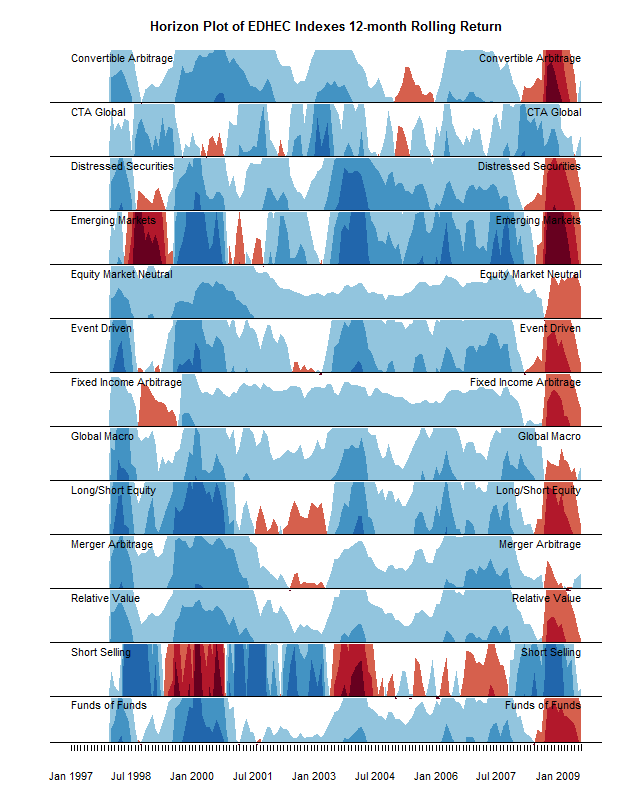Want to share your content on R-bloggers? click here if you have a blog, or here if you don't.

The Timely Portfolio blog via R-bloggers has recently published some interesting entries about the value of horizon plots for visual comparison of a number of time series.Very nice it looks too. You can read more about them here. The trick to understanding them is to imagine that each row was orginally a line chart of six times the height. First colour the area between the origin and the line so that dark reds are very negative and dark blues very positive. Then, for each row, slice the chart into six horizontal slices and lay them on top of one another. That way you save a lot of vertical space so comparisons are easier. Dark red still means very negative, etc. The vertical scale is the same for each chart.

This one was done using the latticeExtra package in R. I couldn’t figure out how to do them in ggplot. It was trivially easy to do a normal line chart and add a coloured background:

```library(ggplot2)
library(reshape)
require(PerformanceAnalytics)
data(edhec)
ed=data.frame(edhec)
ed\$date=as.Date(rownames(ed))
m=melt(ed,id="date")
m\$variable=gsub('\\.',' ',m\$variable)

That’s it.Not as sophisticated, but actually the colours help quite nicely for comparison without the complexity of the horizon approach. It seems less exciting – but then perhaps the horizon plot overstates its case a bit. And it is trivial to understand, which the horizon plot isn’t.

What do you think, is the horizon plot worth the extra effort?
Is there an easy way to do horizon plots in ggplot?